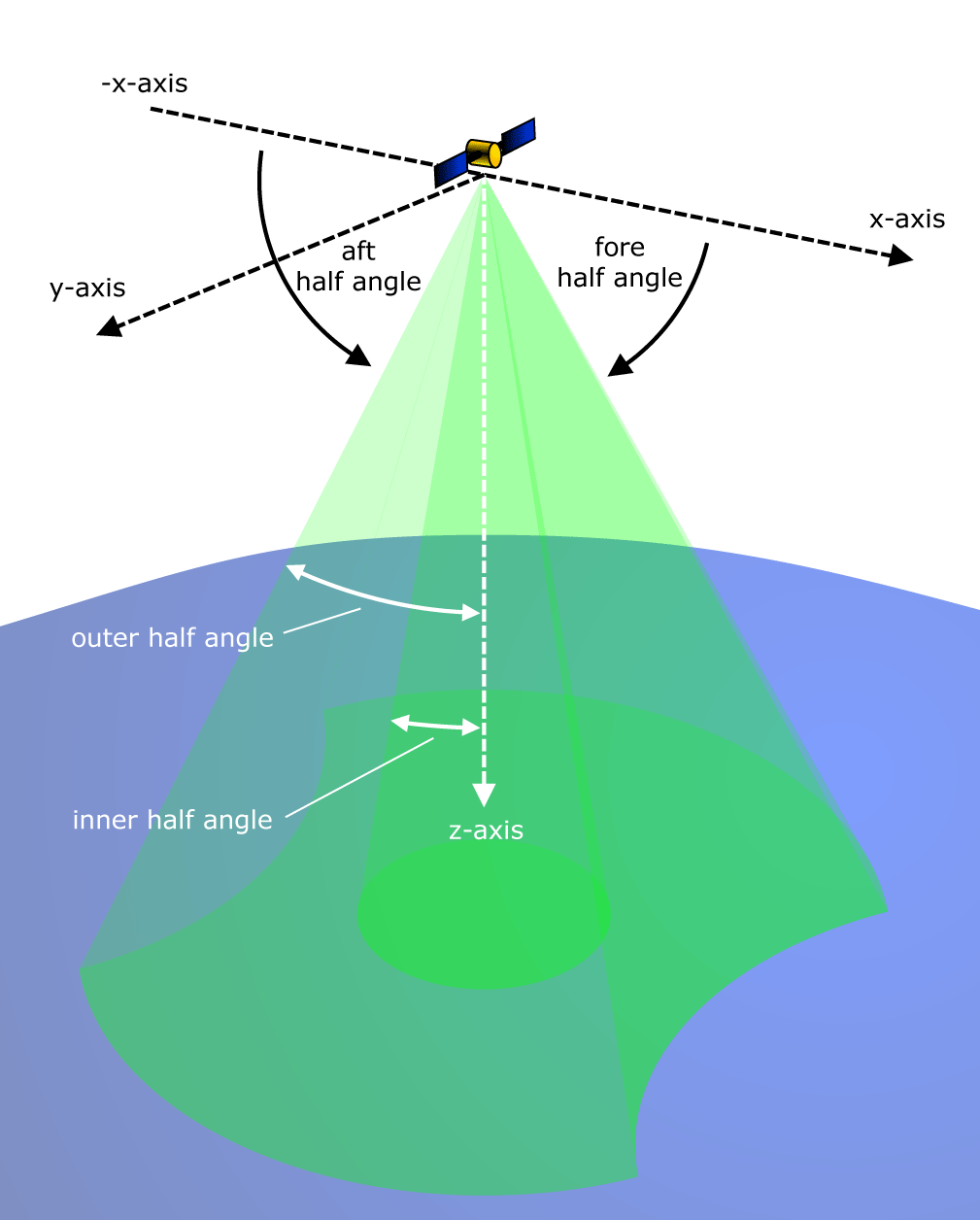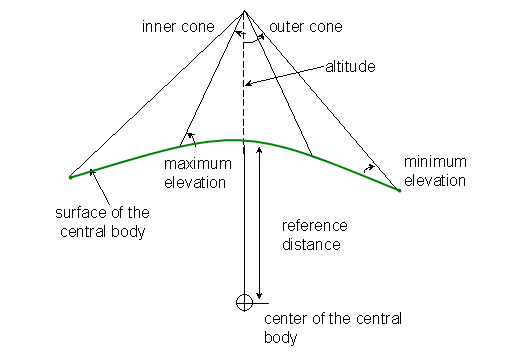agi.foundation.geometry.shapes
(agi.foundation.core-2022r2.jar)

• All Implemented Interfaces:
IServiceProvider

```public class SyntheticApertureRadarVolume
extends SensorFieldOfView```
A sensor volume defined by inner and outer half angles, fore and aft exclusion angles, and a radial range limit. The outer half angle defines the outer boundary of the volume, and the other half angles define exclusion boundaries for the sensor volume.

As depicted in the figure below, the vertex of the volume is located at the origin and the sensor principal direction is along the positive z-axis. Half angles measured from the principal direction are used to define the outer boundary of the volume as well as an interior conical volume of exclusion. Fore and aft half angles are used to define a conical volumes of exclusion about the positive and negative x-axis, respectively. The range limit measured radially from the origin closes the volume.• ### Constructor Summary

Constructors
Constructor and Description
`SyntheticApertureRadarVolume()`
Constructs a sensor volume with a default definition, in which the `InnerHalfAngle` (`get`) has a value of one-fourth pi radians, the `OuterHalfAngle` (`get`) has a value of `Constants.HalfPi` radians, the `ForeHalfAngle` (`get` / `set`) and `AftHalfAngle` (`get` / `set`) are both equal to zero, and the radius is equal to positive infinity.
```SyntheticApertureRadarVolume(double innerHalfAngle, double outerHalfAngle, double aftHalfAngle, double foreHalfAngle)```
Constructs a volume of infinite radius with the provided parameters.
```SyntheticApertureRadarVolume(double innerHalfAngle, double outerHalfAngle, double aftHalfAngle, double foreHalfAngle, double radius)```
Constructs a volume of limited radius with the provided parameters.
• ### Method Summary

All Methods
Modifier and Type Method and Description
`boolean` `encloses(Cartesian point)`
Indicates if the provided point is inside the volume.
`double` `getAftHalfAngle()`
Gets the aft half angle defining the conical volume of exclusion about the negative x-axis.
`EnclosureDescriptionCollection` `getEnclosureDescriptions()`
Gets the list of descriptions for the enclosure functions for this solid.
`double` `getForeHalfAngle()`
Gets the fore half angle defining the conical volume of exclusion about the positive x-axis.
`double` `getInnerHalfAngle()`
Gets the inner half angle used to define the interior conical volume of exclusion.
`boolean` `getIsConnected()`
Gets a value indicating whether the figure is connected.
`double` `getOuterHalfAngle()`
Gets the outer half angle used to define the exterior conical boundary of the volume.
`SensorProjection` ```getProjection(Ellipsoid ellipsoid, KinematicTransformation ellipsoidToSensorTransformation, SensorProjectionOptions projectionOptions)```
Gets the projection of the sensor onto the front surface of a specified ellipsoid and onto a plane at a specified distance in space.
`protected void` `onRadiusChanged()`
Called after the `Radius` (`get` / `set`) property changes.
`void` `setAftHalfAngle(double value)`
Sets the aft half angle defining the conical volume of exclusion about the negative x-axis.
`void` `setForeHalfAngle(double value)`
Sets the fore half angle defining the conical volume of exclusion about the positive x-axis.
`void` ```setInnerOuterHalfAngles(double inner, double outer)```
Sets the inner and outer half angles of a sensor, which define part of the sensor volume.
• ### Methods inherited from class agi.foundation.geometry.shapes.SensorFieldOfView

`getProjection, getRadius, getService, setRadius`
• ### Methods inherited from class java.lang.Object

`clone, equals, finalize, getClass, hashCode, notify, notifyAll, toString, wait, wait, wait`
• ### Constructor Detail

```public SyntheticApertureRadarVolume(double innerHalfAngle,
double outerHalfAngle,
double aftHalfAngle,
double foreHalfAngle)```
Constructs a volume of infinite radius with the provided parameters.
Parameters:
`innerHalfAngle` - The angle between the principal direction and the outermost boundary of the interior conical volume of exclusion. Defines the interior conical volume of exclusion.
`outerHalfAngle` - The angle between the principal direction and the outermost boundary of the sensor volume. Defines the exterior conical boundary of volume.
`aftHalfAngle` - Defines the conical volume of exclusion about the negative x-axis.
`foreHalfAngle` - Defines the conical volume of exclusion about the positive x-axis.

```public SyntheticApertureRadarVolume(double innerHalfAngle,
double outerHalfAngle,
double aftHalfAngle,
double foreHalfAngle,
Constructs a volume of limited radius with the provided parameters.
Parameters:
`innerHalfAngle` - The angle between the principal direction and the outermost boundary of the interior conical volume of exclusion. Defines the interior conical volume of exclusion.
`outerHalfAngle` - The angle between the principal direction and the outermost boundary of the sensor volume. Defines the exterior conical boundary of volume.
`aftHalfAngle` - Defines the conical volume of exclusion about the negative x-axis.
`foreHalfAngle` - Defines the conical volume of exclusion about the positive x-axis.
`radius` - The radial limit of the volume. This is the maximum range at which the sensor can detect a target.
• ### Method Detail

• #### getIsConnected

`public boolean getIsConnected()`
Gets a value indicating whether the figure is connected.
Specified by:
`getIsConnected` in class `Figure`

`protected void onRadiusChanged()`
Called after the `Radius` (`get` / `set`) property changes.
Overrides:
`onRadiusChanged` in class `SensorFieldOfView`
• #### getInnerHalfAngle

`public final double getInnerHalfAngle()`
Gets the inner half angle used to define the interior conical volume of exclusion.
• #### getOuterHalfAngle

`public final double getOuterHalfAngle()`
Gets the outer half angle used to define the exterior conical boundary of the volume.
• #### getForeHalfAngle

`public final double getForeHalfAngle()`
Gets the fore half angle defining the conical volume of exclusion about the positive x-axis.
Throws:
`ArgumentOutOfRangeException` - Thrown when the provided fore half angle is less than zero or greater than or equal to `Constants.HalfPi` radians.
• #### setForeHalfAngle

`public final void setForeHalfAngle(double value)`
Sets the fore half angle defining the conical volume of exclusion about the positive x-axis.
Throws:
`ArgumentOutOfRangeException` - Thrown when the provided fore half angle is less than zero or greater than or equal to `Constants.HalfPi` radians.
• #### getAftHalfAngle

`public final double getAftHalfAngle()`
Gets the aft half angle defining the conical volume of exclusion about the negative x-axis.
Throws:
`ArgumentOutOfRangeException` - Thrown when the provided aft half angle is less than zero or greater than or equal to `Constants.HalfPi` radians.
• #### setAftHalfAngle

`public final void setAftHalfAngle(double value)`
Sets the aft half angle defining the conical volume of exclusion about the negative x-axis.
Throws:
`ArgumentOutOfRangeException` - Thrown when the provided aft half angle is less than zero or greater than or equal to `Constants.HalfPi` radians.
• #### setInnerOuterHalfAngles

```public final void setInnerOuterHalfAngles(double inner,
double outer)```
Sets the inner and outer half angles of a sensor, which define part of the sensor volume.

STK Desktop, unlike STK Components, determines the inner and outer half angles by specifying the maximum and minimum ground elevation angles, respectively. Additionally, a reference radius for the surface of the central body and the altitude of the SAR sensor above the surface must be specified as indicated in the following diagram.Parameters:
`inner` - The angle between the principal direction and the outermost boundary of the interior conical volume of exclusion. Defines the interior conical volume of exclusion.
`outer` - The angle between the principal direction and the outermost boundary of the sensor volume. Defines the exterior conical boundary of volume.
Throws:
`ArgumentOutOfRangeException` - Thrown when either the `inner` or `outer` half angle is outside of the range from zero to pi radians.
`ArgumentException` - Thrown when the `inner` half angle is greater than or equal to the `outer` half angle.
• #### encloses

```public boolean encloses(@Nonnull
Cartesian point)```
Indicates if the provided point is inside the volume.

The `point` parameter must be expressed in the same coordinate frame that the volume is defined in.

Specified by:
`encloses` in class `Solid`
Parameters:
`point` - The point to evaluate.
Returns:
`true` if the point is inside the volume and `false` if the point lies on the boundary or outside of the volume.
• #### getEnclosureDescriptions

`public EnclosureDescriptionCollection getEnclosureDescriptions()`
Gets the list of descriptions for the enclosure functions for this solid.
Specified by:
`getEnclosureDescriptions` in class `Solid`
Returns:
The list of enclosure descriptions.
• #### getProjection

```public SensorProjection getProjection(Ellipsoid ellipsoid,
@Nonnull
KinematicTransformation ellipsoidToSensorTransformation,
SensorProjectionOptions projectionOptions)```
Gets the projection of the sensor onto the front surface of a specified ellipsoid and onto a plane at a specified distance in space.
Specified by:
`getProjection` in class `SensorFieldOfView`
Parameters:
`ellipsoid` - The ellipsoid on which the sensor is to be projected.
`ellipsoidToSensorTransformation` - The transformation from the reference frame of the ellipsoid to the reference frame of the sensor.
`projectionOptions` - The options controlling how the sensor is projected, or `null` to use defaults.
Returns:
The sensor projection.